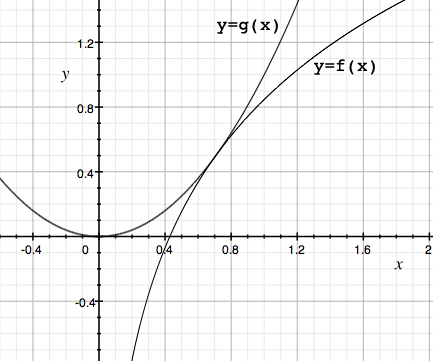SEARCH HOMEMath Central Quandaries & QueriesQuestion from Mike: Prove that y=lnx+(1+ln2)/2 and y=x^2 touch each other. The course is about logarithm and root functions... how should I solve this problem?Hi Mike,

I wasn't sure what was meant by "touch each other" so I plotted the two functions

$f(x) = \ln(x) + \frac{1 + \ln(2)}{2} \mbox{ and } g(x) = x^{2}.$It does look like the two curves have a common tangent at approximately x = 0.7. If they do have a common tangent there then at this point we would have

$f^\prime (x) = g^\prime (x).$

Can you finish it?
PennyMath Central is supported by the University of Regina and The Pacific Institute for the Mathematical Sciences.